Next: Hierarchical clustering Up: Flat clustering Previous: References and further reading   Contents   Index

# Exercises

Exercises.

• Let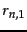be a clustering that exactly reproduces a class structure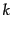and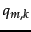a clustering that further subdivides some clusters in. Show that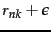.

• Show that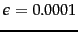.

• Mutual information is symmetric in the sense that its value does not change if the roles of clusters and classes are switched: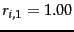. Which of the other three evaluation measures are symmetric in this sense?

• Compute RSS for the two clusterings in Figure 16.7 .

• (i) Give an example of a set of points and three initial centroids (which need not be members of the set of points) for which 3-means converges to a clustering with an empty cluster. (ii) Can a clustering with an empty cluster be the global optimum with respect to RSS?

• Download Reuters-21578. Discard documents that do not occur in one of the 10 classes acquisitions, corn, crude, earn, grain, interest, money-fx, ship, trade, and wheat. Discard documents that occur in two of these 10 classes. (i) Compute a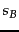-means clustering of this subset into 10 clusters. There are a number of software packages that implement-means, such as WEKA (Witten and Frank, 2005) and R (R Development Core Team, 2005). (ii) Compute purity, normalized mutual information,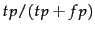and RI for the clustering with respect to the 10 classes. (iii) Compile a confusion matrix (Table 14.5 , page 14.5 ) for the 10 classes and 10 clusters. Identify classes that give rise to false positives and false negatives.

• Prove that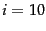is monotonically decreasing in.

• There is a soft version of-means that computes the fractional membership of a document in a cluster as a monotonically decreasing function of the distance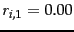from its centroid, e.g., as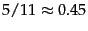. Modify reassignment and recomputation steps of hard-means for this soft version.

• In the last iteration in Table 16.3 , document 6 is in cluster 2 even though it was the initial seed for cluster 1. Why does the document change membership?

• The values of the parameters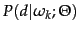in iteration 25 in Table 16.3 are rounded. What are the exact values that EM will converge to?

• Perform a-means clustering for the documents in Table 16.3 . After how many iterations does-means converge? Compare the result with the EM clustering in Table 16.3 and discuss the differences.

• Modify the expectation and maximization steps of EM for a Gaussian mixture. The maximization step computes the maximum likelihood parameter estimates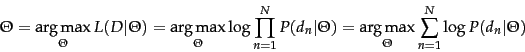,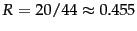, and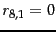for each of the clusters. The expectation step computes for each vector a soft assignment to clusters (Gaussians) based on their current parameters. Write down the equations for Gaussian mixtures corresponding to and 202 .

• Show that-means can be viewed as the limiting case of EM for Gaussian mixtures if variance is very small and all covariances are 0.

• The within-point scatter of a clustering is defined as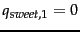. Show that minimizing RSS and minimizing within-point scatter are equivalent.

• Derive an AIC criterion for the multivariate Bernoulli mixture model from Equation 196.Next: Hierarchical clustering Up: Flat clustering Previous: References and further reading   Contents   Index